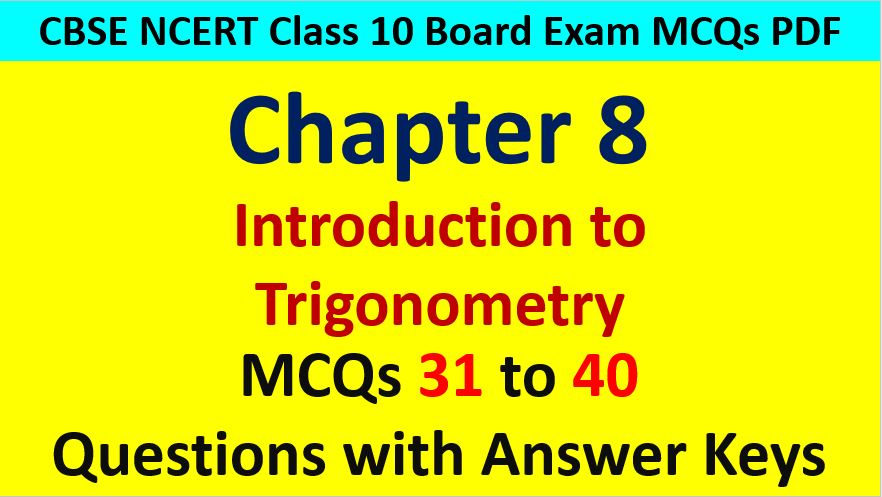Friday, October 22, 2021
Home > CBSE Class 10 > Extra MCQ Questions for Class 10 Maths Chapter 8 Trigonometry

# Extra MCQ Questions for Class 10 Maths Chapter 8 TrigonometryHi students, Welcome to AMBiPi (Amans Maths Blogs). In this article, you will get Extra MCQ Questions for Class 10 Maths Chapter 8 Trigonometry. You can download this PDF and save it in your mobile device or laptop etc.

Extra MCQ Questions for Class 10 Maths Trigonometry Question No 31:

The value of tanθ/(secθ – 1) + tanθ/(secθ + 1) is equal to

Option A : 2tanθ

Option B : 2secθ

Option C : 2cosecθ

Option D : 2tanθsecθ

Option C : 2cosecθ

Extra MCQ Questions for Class 10 Maths Trigonometry Question No 32:

If x = acosθ and y = bsinθ, then the value of b2x2 + a2y2 is

Option A : a2b2

Option B : ab

Option C : a4b4

Option D : a2 + b2

Option A : a2b2

Extra MCQ Questions for Class 10 Maths Trigonometry Question No 33:

If x = asecθ and y = btanθ, then the value of b2x2 – a2y2 is

Option A : ab

Option B : a2 – b2

Option C : a2 + b2

Option D : a2b2

Option D : a2b2

Extra MCQ Questions for Class 10 Maths Trigonometry Question No 34:

(1 + tanθ + secθ)(1 + cotθ – cosecθ) is equal to

Option A : 0

Option B : 1

Option C : 2

Option D : -1

Option C : 2

Extra MCQ Questions for Class 10 Maths Trigonometry Question No 35:

The value of (secA + tanA)(1 – sinA) is equal to

Option A : secA

Option B : sinA

Option C : cosecA

Option D : cosA

Option D : cosA

Extra MCQ Questions for Class 10 Maths Trigonometry Question No 36:

The value of (1 + tan2A)/(1 + cot2A) is equal to

Option A : sec2A

Option B : -1

Option C : cot2A

Option D : tan2A

Option D : tan2A

Extra MCQ Questions for Class 10 Maths Trigonometry Question No 37:

The value of (cos4x – sin4x) is equal to

Option A : 2sin2x – 1

Option B : 1 – 2cos2x

Option C : 2sin2x – cosx

Option D : 2cos2x – 1

Option D : 2cos2x – 1

Extra MCQ Questions for Class 10 Maths Trigonometry Question No 38:

If tanθ + sinθ = m and tanθ  sinθ = n, then the value of m2 – n2 is

Option A : √mn

Option B : √m/n

Option C : 4√mn

Option D : None of these

Option C : 4√mn

Extra MCQ Questions for Class 10 Maths Trigonometry Question No 39:

If 7sin2θ + 3cos2θ = 4, then the value of secθ + cosecθ is equal to

Option A : 2/√3 – 2

Option B : 2/√3 + 2

Option C : 2/√3

Option D : None of these

Option B : 2/√3 + 2

Extra MCQ Questions for Class 10 Maths Trigonometry Question No 40:

If acosθ + bsinθ = 4 and asinθ – bcosθ = 3, then the value of a2 + b2 is

Option A : 7

Option B : 12

Option C : 25

Option D : None of these